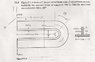# Radial stress in curved beams

• Jonny Black

#### Jonny Black

I have a u-shaped t-beam, and I am trying to calculate the radial stress where $$\theta$$=0 degrees. I have calculated $$\sigma\theta_{}$$ but I am unclear on how to treat the varying thickness of the cross-section when integrating with respect to radius.

#### Attachments

•scan0035.jpg
38.1 KB · Views: 1,114

Hey Johnny,

You have to show your work first, so we can pinpoint where you went wrong.

$$\sigma_{r}=\frac{1}{tr}\int t\sigma_{\theta}dr$$
with a lower limit of a=inner radius, and upper limit of r=variable radius. For one, why is the thickness even included in the equation since it cancels anyway, and two, how do I treat the varying thickness of the cross-section? I have tried
$$\sigma_{r}=\frac{1}{t_{1}r}\int^{b}_{a} t_{1}\sigma_{\theta}dr+\frac{1}{t_{2}r}\int^{c}_{b} t_{2}\sigma_{\theta}dr$$

$$\sigma_{r}=\frac{1}{t_{1}r}\int^{r}_{a} t_{1}\sigma_{\theta}dr+\frac{1}{t_{2}r}\int^{r}_{b} t_{2}\sigma_{\theta}dr$$
where the subscripts 1 & 2 denote the horizontal and vertical portions of the cross-section, respectively. Neither method gives viable results. a, b, and c denote radius's at each definition of the cross-section starting with the inner radius. I have found $$\sigma_{\theta}$$ already, I just need to know how to define the limits of the integral

I have added a couple of files for clarification. Also, I am plotting the radial stress as a function of the radius so the end result will include the variable "r".

...Anybody...?